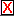Home > JavaSketchpad > Gallery > Sine Wave Geometry

# Sine Wave Geometry

In the illustration below, X is a point on the yellow circle, and time is a point on the horizontal segment (axis). Drag each of these points with the mouse.

The red line passing horizontally through X isolates the height of X above (or below) the axis. This "height" of a point on a unit circle is known as the sine of the angle determined by that point (from the center of the circle). Thus, the red line represents sin(X) geometrically. As you drag X around the circle, sin(X) rises and falls.

The blue line passing vertically through time simply represents a single moment of time. As you drag time along its axis, the given moment of time is "earlier" (to the left) or "later" (to the right).

The point P falls at the intersection of the red and blue lines. It, therefore, represents the sine of X (because it falls on the red line) at a given moment in time (because it falls on the blue line). As you drag things, this point leaves behind a trace of where it has been.

You can clear these traces whenever you want by clicking the.

Sorry, this page requires a Java-compatible web browser.

Now look at what happens to P when you move X around the circle while moving time along its axis simultaneously. Because your computer doesn't have two mice, you can't drag these both at once, so you'll have to use the buttons at the bottom of the JavaSketch. Press Spin X to start moving X around its circle, and then press Increase Time to move time along its axis.

The resulting curve is the sine wave, which plots the height of a point moving around the unit circle as a function of time.

• If you could measure geometric quantities in this JavaSketch numerically, how would you compute the value of the sine function for a given position of X?
• What would happen if time moved slower than X? If X moved slower than time?
• Notice, when time reaches the end of its axis, that it starts a new sine wave in a different place than the sine wave it just finished plotting. How could you change this construction so that it only produces one sine wave, no matter how long the points keep moving? (Drag objects to test your answer.)

Here's a more complex construction. It was created by placing many points X all around the circle, and many points time all along the axis. Each horizontal line through each X intersects exactly one vertical line through a point time, resulting in many points simultaneously falling at different places along the sine curve. These points are then connected with blue regions. Pressing the Spin button causes all of the points X to rotate around the circle at the same rate. You can use the other buttons to reveal the rest of the construction.

Sorry, this page requires a Java-compatible web browser.